Next: 4.3.1 Newton's Method Up: 4. Numerical Implementation Previous: 4.2.4 Discretization of the

# 4.3 Simulation in FEDOS

FEDOS (Finite Element Diffusion and Oxidation Simulator) is a finite element based framework for simulating fabrication processes to be used in the microelectronics industry [155,156]. It integrates the basic functionality required for finite element analysis, namely, assembly routines, linear and non-linear solvers, input and output operations, etc, so that the equations derived in Section 4.2 can be conveniently implemented.

Since electromigration constitutes a multi-physics problem, it is convenient to divide it into smaller subproblems and to solve each one separately. The resulting simulation scheme is depicted in Figure 4.3. The electro-thermal problem is governed by partial differential equations for the electric potential,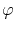, and for the temperature,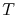. The variables of interest for the vacancy dynamics are the vacancy concentration,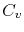, and the trapped vacancy concentration at grain boundaries and interfaces,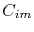. The mechanical problem is formulated in terms of the displacement field,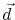, however, the mechanical stress obtained from the solution of the mechanical problem is the variable of interest here. Each subproblem is solved for the corresponding variable, while the variables of the other subproblems are fixed.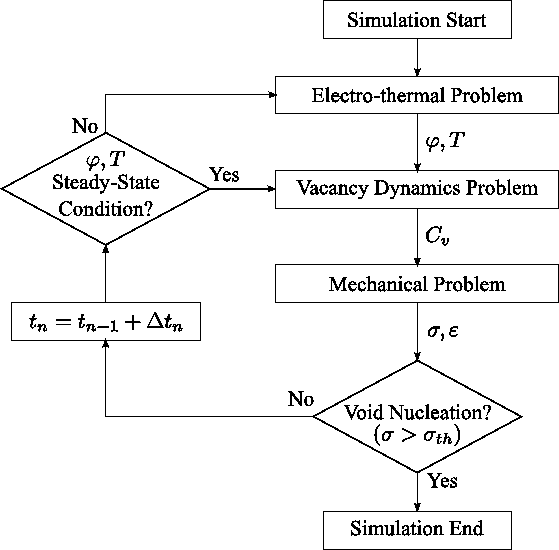First, the solution of the electro-thermal problem determines the electric potential and temperature distribution in the interconnect. These quantities are then used to compute the vacancy concentration change due to the total mass transport occurring under electromigration and accompanying driving forces. The rate of change of the trapped vacancy concentration at grain boundaries and other interfaces due to generation/annihilation processes is obtained. The change in vacancy concentration determines the induced strain which loads the mechanical problem. The solution of the mechanical system yields the deformation of the interconnect line, so that the mechanical stress can be calculated. If the magnitude of the developed stress reaches the threshold value for void nucleation, the simulation procedure is terminated and the corresponding time for void nucleation, and eventually interconnect failure, can be estimated. Otherwise, the time is updated and the cycle described above is repeated. Since the electric potential and temperature distribution reach a steady state condition in a time scale much shorter than that for the vacancy concentration and mechanical stress, the electro-thermal problem is typically solved only for a few initial time steps.

All subproblems are solved on the same finite element mesh. An initial mesh refinement routine may be executed in the beginning of the simulation procedure in order to appropriately solve the material transport equations at grain boundaries and material interfaces.

SubsectionsNext: 4.3.1 Newton's Method Up: 4. Numerical Implementation Previous: 4.2.4 Discretization of the

R. L. de Orio: Electromigration Modeling and Simulation